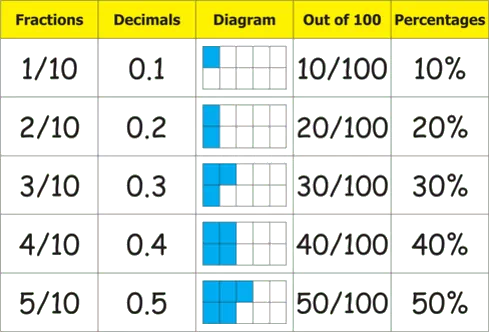# Fractions and Decimals worksheet for class 7## myCBSEguide App

CBSE, NCERT, JEE Main, NEET-UG, NDA, Exam Papers, Question Bank, NCERT Solutions, Exemplars, Revision Notes, Free Videos, MCQ Tests & more.

CBSE worksheets for Fractions and Decimals worksheet for class 7 in PDF for free download. Mathematics worksheets for class 7 CBSE includes worksheets on Fractions and Decimals as per NCERT syllabus. CBSE class 7 worksheets as PDF for free download Fractions and Decimals worksheets. Users can download and print the worksheets on class 7 Mathematics Fractions and Decimals for free.

# Download Fractions and Decimals worksheet for class 7

## Fractions and Decimalsworksheet for class 7 Important Topics

• Let’s recal fractions
• Multiplication of Fractions (fraction x whole number & fractionx fraction)
• Division of fractions (whole number/fraction; reciprocal of a fraction; fraction/whole number; fraction/fraction)
• How Well have you learnt about decimal numbers
• Multiplication & Division of Decimal Numbers (decimal x decimal; decimal x whole number; decimal x 10, 100, 1000)
• Word Problems on Fractions and Decimal Numbers## Some important Facts about Fractions and Decimalsworksheet for class 7

1. We have learnt about fractions and decimals alongwith the operations of addition and subtraction on them, in the earlier class.
2. We now study the operations of multiplication and division on fractions as well as on decimals.
3. We have learnt how to multiply fractions. Two fractions are multiplied by multiplying their numerators and denominators seperately and writing the product as product of numerators product of denominators . For example, 2 5 2 × 5 10 × 3 7 3× 7 21 .
4.  We have seen how to divide two fractions.
1. While dividing a whole number by a fraction, we multiply the whole number with the reciprocal of that fraction.

## NCERT class 7Mathematics Solved Worksheets

• Chapter 1 – Integers
• Chapter 2 – Fractions and Decimals
• Chapter 3 – Data Handling
• Chapter 4 – Simple Equations
• Chapter 5 – Lines and Angles
• Chapter 6 – Practical Geometry
• Chapter 7 – The Triangle and its Properties
• Chapter 8 – Congruence of triangles
• Chapter 9 – Comparing Quantities
• Chapter 10 – Rational numbers
• Chapter 11 – Perimeter and Area
• Chapter 12 – Algebraic Expressions
• Chapter 13 – Exponents and Powers
• Chapter 14 – Symmetry
• Chapter 15 – Visualizing Solid Shapes

## MULTIPLICATION OF DECIMAL NUMBERS

Reshma purchased 1.5kg vegetable at the rate of  8.50 per kg. How much money should she pay? Certainly it would be  (8.50 × 1.50). Both 8.5 and 1.5 are decimal numbers. So, we have come across a situation where we need to know how to multiply two decimals. Let us now learn the multiplication of two decimal numbers.

To download Printable worksheets for class 7 Mathematics and Science; do check myCBSEguide app or website. myCBSEguide provides sample papers with solution, test papers for chapter-wise practice, NCERT solutions, NCERT Exemplar solutions, quick revision notes for ready reference, CBSE guess papers and CBSE important question papers. Sample Paper all are made available through the best app for CBSE students and myCBSEguide website.### 1 thought on “Fractions and Decimals worksheet for class 7”

1. Make test paper of maths for class 7 integers and fractions and decimal